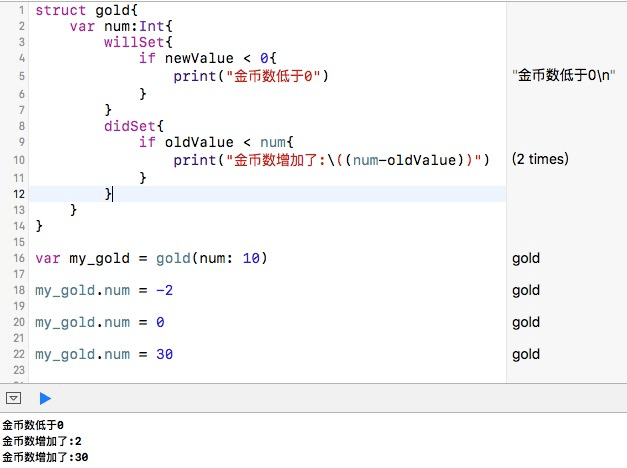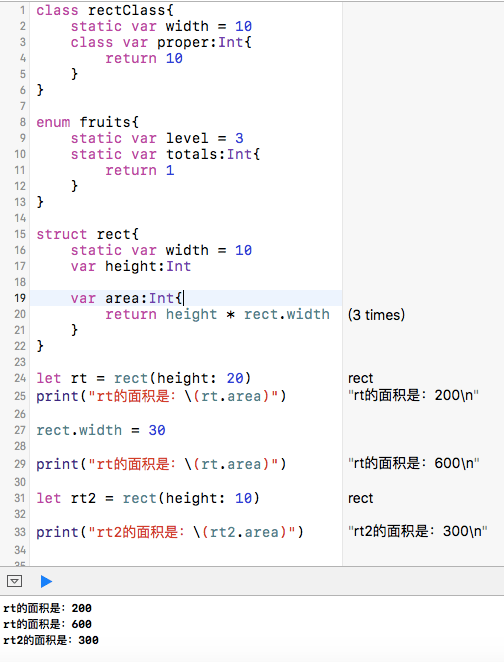# 存储属性

``````struct size {
var width:Int
let height:Int
}

var s_var = size(width: 10, height: 20)
s_var.width = 30
//s_var.height = 40，无法编译，常量属性，不能被改变

let s_constant = size(width: 50, height: 60)
//d_var.width = 60，无法编译，常量结构体所有属性只能被初始化一次
``````

# 计算属性

``````struct rect {
var width:Int
let height:Int
var circumference:Int{
get{
return 2*(width+height)
}
set(circu){
width = circu/2 - height
}
}
}

var r = rect(width: 20, height: 10)

print("现在的周长是：\(r.circumference)")
//打印结果：现在的周长是：60
r.circumference = 50

print("更改后宽度是:\(r.width)，周长是\(r.circumference)")
//打印结果：更改后宽度是:15，周长是50

``````

``````    var circumference:Int{
get{
return 2*(width+height)
}
set{
width = newValue/2 - height
}
}
``````

``````struct rect {
var width:Int
let height:Int
var area:Int{
return width*height
}
}

var r = rect(width: 20, height: 10)

print("现在的面积是：\(r.area)")
//现在的面积是：200
``````

# 属性监视器

willSet和didSet都可以设置一个常量形参来传递新、旧属性值。常量形参如果不设置，则使用默认的newValue、oldValue来传递。

``````struct gold{
var num:Int{
willSet{
if newValue < 0{
print("金币数低于0")
}
}
didSet{
if oldValue < num{
print("金币数增加了:\((num-oldValue))")
}
}
}
}

var my_gold = gold(num: 10)

my_gold.num = -2

my_gold.num = 0

my_gold.num = 30
``````# 类型属性

``````class rectClass{
static var width = 10
class var proper:Int{
return 10
}
}

enum fruits{
static var level = 3
static var totals:Int{
return 1
}
}

struct rect{
static var width = 10
var height:Int

var area:Int{
return height * rect.width
}
}

let rt = rect(height: 20)
print("rt的面积是：\(rt.area)")

rect.width = 30

print("rt的面积是：\(rt.area)")

let rt2 = rect(height: 10)

print("rt2的面积是：\(rt2.area)")
``````# 示例代码

https://github.com/99ios/23.12